# Exponentiation + multiplication - math problems

If n is a positive integer and x is any real number, then x^n corresponds to repeated multiplication x^n=x×x×⋯×x (n times). We can call this “x raised to the power of n,” “x to the power of n,” or simply “x to the n.” Here, x is the base, and n is the exponent or the power.

#### Number of problems found: 18

• Subsets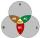How many are all subsets of set ??
• Bits, bytes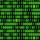Calculate how many different numbers can be encoded in 16-bit binary word?
• Compare IIWhich of the numbers 710, 107 is greater?
• Area codes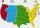How many 6 digit area codes are possible if the first number can't be zero?
• Events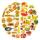Event P has probability of 0.84. What is the probability that the event P occurs in 3, 5, 7 try.
• Morse alphabet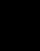Calculate how many words of Morse code to create compiling dashes and dots in the words of one to four characters.
• No. of divisorsHow many different divisors have number ??
• InterestCalculate how much you earn for 10 years 43000 deposit if the interest rate is 1.3% and the interest period is a quarter.
• Ray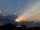Light ray loses 1/19 of brightness passing through glass plate. What is the brightness of the ray after passing through 7 identical plates?
• JUDr. Usury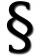Judge JUDr. Usury approved the agreement on guilt and punishment where confessed to pay daily interest 0.18%. How big is a yearly interest? Year has 360 days.
• Gp - 80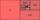Sum of the first four members of a geometric progression is 80. Determine they if we know that the fourth member is nine times greater than the second.
• Boys and girlsThere are eight boys and nine girls in the class. There were six children on the trip from this class. What is the probability that left a) only boys b) just two boys
• Eight palm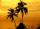There grows 8 palms by the sea. At the first sitting one parrot, on second two, on third sits four parrots on each other twice the previous parrots sitting on a previous palm. How many parrots sitting on eighth palm?
• LoanApply for a \$ 59000 loan, the loan repayment period is 8 years, the interest rate 7%. How much should I pay for every month (or every year if paid yearly). Example is for practise geometric progression and/or periodic payment for an annuity.
• Future of libraries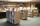You know that thanks to the Internet, electronic communications and developing online resources on the Internet annually declining number of traditional readership by 17%. These deal is irreversible evolutionary shift from old books from libraries to imme
• Cube constructionA 2×2×2 cube is to be constructed using 4 white and 4 black unit cube. How many different cubes can be constructed in this way? ( Two cubes are not different if one can be obtained by rotating the other. )
• Motion problemFrom Levíc to Košíc go car at speed 81 km/h. From Košíc to Levíc go another car at speed 69 km/h. How many minutes before the meeting will be cars 27 km away?
• Profitable bank deposit 2012Calculate the value of what money lose creditor with a deposit € 9500 for 4 years if the entire duration is interest 2.6% p.a. and tax on interest is 19%. Annual inflation is 3.7% (Calculate what you will lose if you leave money lying idle at negative int

Do you have an interesting mathematical word problem that you can't solve it? Submit a math problem, and we can try to solve it.

We will send a solution to your e-mail address. Solved examples are also published here. Please enter the e-mail correctly and check whether you don't have a full mailbox.

Please do not submit problems from current active competitions such as Mathematical Olympiad, correspondence seminars etc...